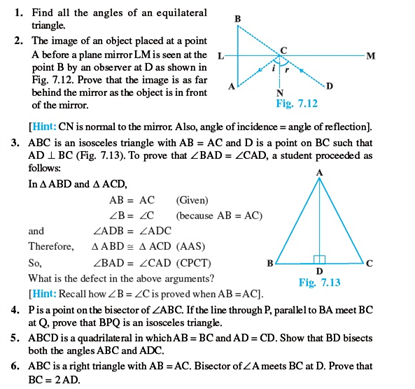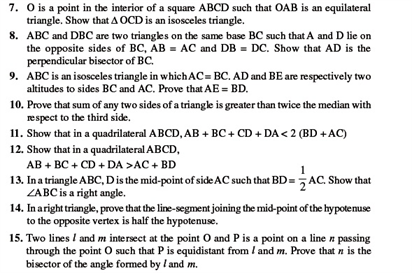# Revision Notes For CBSE Class 9 Math Chapter -7 Triangles

A triangle is a plane figure with three straight sides and three angles. The sum of angles of a triangle is equal to 180 degrees and the exterior angles of a triangle are always equal to 360 degrees. In a triangle, the sum of the angles is always greater than the length of the third angle. The area of a triangle is given by the formulae:

½ * base * height.

A triangle is classified into different types based on their sides and angles.

• Equilateral Triangle
• Isosceles triangle
• Scalene Triangle
• Acute angled Triangle
• Right angle Triangle
• Obtuse-angled Triangle

Check here for the free CBSE Class 9 Math revision notes for Chapter 7- Triangles